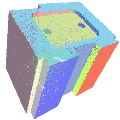CGAL 5.0.2 - Shape Detection
Shape Detection ReferenceSven Oesau, Yannick Verdie, Clément Jamin, Pierre Alliez, Florent Lafarge, Simon Giraudot, Thien Hoang, and Dmitry Anisimov
This CGAL package implements the Efficient RANSAC (RANdom SAmple Consensus) approach for detecting arbitrary shapes in an unorganized point set with unoriented normals and the Region Growing approach for detecting shapes in a set of arbitrary items. With the Efficient RANSAC approach, five canonical shapes can be detected: planes, spheres, cylinders, cones, and tori. Additional shapes can be detected, given a custom shape class by the user. For the Region Growing approach, CGAL provides three particular shape detection components: detecting lines in a 2D point set, detecting planes in a 3D point set, and detecting planes on a polygon mesh.
Introduced in: CGAL 4.7
BibTeX: cgal:ovja-pssd-20a
Windows Demo: Polyhedron demo
Common Demo Dlls: dlls

## Efficient RANSAC

### Concepts

• EfficientRANSACTraits

### Algorithm

• CGAL::Shape_detection::Efficient_RANSAC_traits<GeomTraits, InputRange, PointMap, NormalMap>
• CGAL::Shape_detection::Efficient_RANSAC<Traits>

### Shapes

• CGAL::Shape_detection::Plane<Traits>
• CGAL::Shape_detection::Sphere<Traits>
• CGAL::Shape_detection::Cylinder<Traits>
• CGAL::Shape_detection::Cone<Traits>
• CGAL::Shape_detection::Torus<Traits>

### Shape Interface

• CGAL::Shape_detection::Shape_base<Traits>

### Property Maps

• CGAL::Shape_detection::Plane_map<Traits>
• CGAL::Shape_detection::Point_to_shape_index_map<Traits>

## Region Growing

### Concepts

• NeighborQuery
• RegionType

### Algorithm

• CGAL::Shape_detection::Region_growing<InputRange, NeighborQuery, RegionType, SeedMap>

### Point Set

• CGAL::Shape_detection::Point_set::K_neighbor_query<GeomTraits, InputRange, PointMap>
• CGAL::Shape_detection::Point_set::Sphere_neighbor_query<GeomTraits, InputRange, PointMap>
• CGAL::Shape_detection::Point_set::Least_squares_line_fit_region<GeomTraits, InputRange, PointMap, NormalMap>
• CGAL::Shape_detection::Point_set::Least_squares_plane_fit_region<GeomTraits, InputRange, PointMap, NormalMap>
• CGAL::Shape_detection::Point_set::Least_squares_line_fit_sorting<GeomTraits, InputRange, NeighborQuery, PointMap>
• CGAL::Shape_detection::Point_set::Least_squares_plane_fit_sorting<GeomTraits, InputRange, NeighborQuery, PointMap>

### Polygon Mesh

• CGAL::Shape_detection::Polygon_mesh::One_ring_neighbor_query<PolygonMesh, FaceRange>
• CGAL::Shape_detection::Polygon_mesh::Least_squares_plane_fit_region<GeomTraits, PolygonMesh, FaceRange, VertexToPointMap>
• CGAL::Shape_detection::Polygon_mesh::Least_squares_plane_fit_sorting<GeomTraits, PolygonMesh, NeighborQuery, FaceRange, VertexToPointMap>

## Deprecated Components

• CGAL::Shape_detection::deprecated::Shape_detection_traits<GeomTraits, InputRange, PointMap, NormalMap>
• CGAL::Shape_detection::deprecated::Region_growing_depr<Traits>

## Functions

• CGAL::regularize_planes()

## Modules

Region Growing
Reference Manual for the Region Growing shape detection component.

Efficient RANSAC
Reference Manual for the Efficient RANSAC shape detection component.

Deprecated
Deprecated components.

## Functions

template<typename PointRange , typename PointMap , typename PlaneRange , typename PlaneMap , typename IndexMap , typename Kernel >
void CGAL::regularize_planes (const PointRange &points, PointMap point_map, PlaneRange &planes, PlaneMap plane_map, IndexMap index_map, const Kernel &, bool regularize_parallelism, bool regularize_orthogonality, bool regularize_coplanarity, bool regularize_axis_symmetry, double tolerance_angle=25.0, double tolerance_coplanarity=0.01, typename Kernel::Vector_3 symmetry_direction=typename Kernel::Vector_3(0.0, 0.0, 1.0))
Given a set of detected planes with their corresponding inlier sets, this function enables to regularize the planes: More...

## ◆ regularize_planes()

template<typename PointRange , typename PointMap , typename PlaneRange , typename PlaneMap , typename IndexMap , typename Kernel >
 void CGAL::regularize_planes ( const PointRange & points, PointMap point_map, PlaneRange & planes, PlaneMap plane_map, IndexMap index_map, const Kernel & , bool regularize_parallelism, bool regularize_orthogonality, bool regularize_coplanarity, bool regularize_axis_symmetry, double tolerance_angle = 25.0, double tolerance_coplanarity = 0.01, typename Kernel::Vector_3 symmetry_direction = typename Kernel::Vector_3 (0.0, 0.0, 1.0) )

#include <CGAL/Regularization/regularize_planes.h>

Given a set of detected planes with their corresponding inlier sets, this function enables to regularize the planes:

• Planes near parallel can be made exactly parallel;
• Planes near orthogonal can be made exactly orthogonal;
• Planes parallel and near coplanar can be made exactly coplanar;
• Planes near symmetrical with a user-defined axis can be made exactly symmetrical.

Planes are directly modified. Points are left unaltered, as well as their relationships to planes (no transfer of point from a primitive plane to another).

The implementation follows .

Template Parameters
 PointRange must be a model of ConstRange with points. PointPMap must be a model of ReadablePropertyMap with value type Kernel::Point_3. It can be omitted if the value type of the iterator of PointRange is convertible to Point_3. PlaneRange must be a model of Range with planes. PlaneMap must be a model of WritablePropertyMap with value type Kernel::Plane_3. It can be omitted if the value type of the iterator of PlaneRange is convertible to Plane_3. IndexMap must be a model of ReadablePropertyMap with value type int. Kernel must be a geometric traits class. It can be omitted and deduced automatically from the value type of PointMap.
Parameters
 points ConstRange of points point_map property map: value_type of typename PointRange::const_iterator -> Point_3 planes range of planes plane_map property map: value_type of typename PlaneRange::iterator -> Plane_3 index_map property map: index of point std::size_t -> index of plane int (-1 if the point is not assigned to a plane) regularize_parallelism select whether parallelism is regularized or not regularize_orthogonality select whether orthogonality is regularized or not regularize_coplanarity select whether coplanarity is regularized or not regularize_axis_symmetry select whether axis symmetry is regularized or not tolerance_angle tolerance of deviation between normal vectors of planes (in degrees) used for parallelism, orthogonality, and axis symmetry. Default value is 25 degrees. tolerance_coplanarity maximal distance between two parallel planes such that they are considered coplanar. Default value is 0.01. symmetry_direction chosen axis for symmetry regularization. Default value is the Z axis.
Examples:
Shape_detection/efficient_RANSAC_and_plane_regularization.cpp.